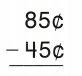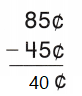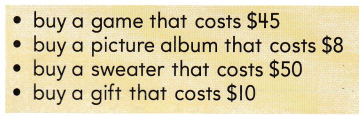# Texas Go Math Grade 2 Lesson 20.4 Answer Key Borrowing Money

Refer to our Texas Go Math Grade 2 Answer Key Pdf to score good marks in the exams. Test yourself by practicing the problems from Texas Go Math Grade 2 Lesson 20.4 Answer Key Borrowing Money.

## Texas Go Math Grade 2 Lesson 20.4 Answer Key Borrowing Money

Essential Question
What does it mean to borrow money?
Explanation:
To obtain or receive (something, such as money) on loan for temporary use, intending to give it, or something equivalent or identical, back to the lender is borrowing.

Explore
Show the addition or subtraction for each problem.

Jackson has $28 in the bank. He makes a deposit of$3.
28 + 3 = 31
if it states as deposit then we have to add.

Now he has ___ in the bank.
Explanation:
Now he has $31 in the bank. Lynn has$23 in the bank. She makes a withdrawal of $3. Answer: 23 – 3 =$20
If it states as withdrawal then we have to subtract

Now she has ___ in the bank.
Explanation:
Now she has $30 in the bank. FOR THE TEACHER • Read each problem with children. Point out that they will need to either add or subtract to solve for the amount. Explanation: if it states as deposit then we have to add. If it states as withdrawal then we have to subtract Math Talk Mathematical Processes Explain how you decided to add or subtract for each problem. Explanation: if it states as deposit then we have to add. If it states as withdrawal then we have to subtract Model and Draw Sometimes people borrow money when they need more money than they have. When you borrow from someone, you plan to give it back to them later.Brian has 45¢. He wants to buy a pen that costs 85¢. How much money does he need to borrow?So, he needs to borrow ___ Explanation:So, he needs to borrow 40¢ Share and Show Solve. Show your work. Question 1. Wendy has 75¢, She wants to buy a snack that costs 90¢. How much money does she need to borrow? Answer: 90 – 75 = 15¢ Explanation: Wendy has 75¢, She wants to buy a snack that costs 90¢. 90 – 75 = 15¢$15 is the money that she need to borrow

Question 2.
Carl has $4. He wants to buy a book that costs$6. How much money does he need to borrow?
6 – 4 =$2 Explanation: Carl has$4. He wants to buy a book that costs $6. 6 – 4 =$2
$2is the money that he need to borrow Question 3. Molly shares her snack with Ted. Is this an example of borrowing? Explain. Answer: No. Explanation: Molly shares her snack with Ted is the example of sharing if he takes and gives in return that is borrowing. Question 4. Describe an example of when you borrowed something. Answer: Diana has to mend a piano she has$10 she need $15 So, the remaining amount she has to take from a person and return it back this is called borrowing. Explanation: 15 – 10 = 5 she has to borrow$5.

Problem Solving

Question 5.
Gino has 63¢. He wants to buy a puzzle that costs 95¢. How much money does he need to borrow?
95 – 63 = 32¢
Explanation:
Gino has 63¢. He wants to buy a puzzle that costs 95¢.
95 – 63 = 32¢
32¢ is the money that he need to borrow

Use the information to solve.

Jenna has $5 today. She can earn$2 each week by helping her mom.

Question 6.
Look at the list of four choices. Which choice is an example of a good reason for Jenna to borrow money? Explain why.Buy a book that costs $6 Explanation: Because remaining are not needs. Question 7. H.O.T. Multi-Step Which of the four choices might be a bad reason for Jenna to borrow money? Explain. Answer: Buy a toy Explanation: 35 is the cost of toy 1 week =$2
35 – 5 = 30
15 x 2 = 30
she  has work for 15 weeks to buy a  toy
Buying a toy might be a bad reason for Jenna to borrow money

How long would it take for Jenna to earn enough to pay back the money that she borrowed for this?
Explanation:
For buying a book she has to work for 1 week to return the money

Question 8.
Wesley wants to buy a game that costs 84¢. He has 59¢. How much money would he need to borrow to buy the game?
(A) 35¢
(B) 48¢
(C) 25¢
Explanation:
Wesley wants to buy a game that costs 84¢. He has 59¢.
84 – 59 = 25¢
25¢ is the money that he need to borrow to buy the game

Question 9.
Describe two examples of borrowing.
Taking and giving back is called borrowing.
Explanation:
Taking money to buy a book
Taking money for some health issues
are some good examples of borrowing

Question 10.
Read the examples of borrowing. Circle the good examples. Draw a line through the bad examples.

• borrowing a pencil and giving it back broken
• borrowing a book and giving it back a few days later
• borrowing an amount of money that will take a very long time to pay back
• borrowing a dollar and paying it back the next day
• borrowing a scarf and washing it before you return itExplanation:

• borrowing a pencil and giving it back broken
• borrowing a book and giving it back a few days later
• borrowing an amount of money that will take a very long time to pay back
• borrowing a dollar and paying it back the next day
• borrowing a scarf and washing it before you return it

Question 11.
Texas Test Prep Andrea has 46¢. She wants to buy a marker that costs 72¢. How much money does she need to borrow?
(A) 26¢
(B) 36¢
(C) 34¢
Explanation:
Andrea has 46¢. She wants to buy a marker that costs 72¢.
72 – 46 = 26
26¢ money that she need to borrow

### Texas Go Math Grade 2 Lesson 20.4 Homework and Practice Answer Key

Question 1.
Gary has 49¢. He wants to buy a marker that costs 85¢. How much money does he need to borrow?
Explanation:
Gary has 49¢. He wants to buy a marker that costs 85¢.
85 – 49 = 36¢
36¢ is the money that he need to borrow

Question 2.
Ellie has $7. She wants to buy a game that costs$12. How much money does she need to borrow?
12 – 7 = $5 Explanation: Ellie has$7. She wants to buy a game that costs $12. 12 – 7 =$5
$5 is the money that she need to borrow Use the information to solve. Kate has$5 today. She can earn $3 each week by taking care of her sister.Question 3. Multi-Step Look at the list of four choices. Which choice is an example of a good reason for Kate to borrow money? Explain. Answer: Buying a sweater is the good example Explanation: The remaining three are not essential Sweater is a need How long would it take for Kate to earn enough to pay back the money that she borrowed for this? Explanation: Kate has 5 50 – 5 = 45 3 per week = 45 divided by 3 = 15 it would take 15 weeks to return back. Lesson Check Texas Test Prep Choose the correct answer. Question 4. Paul has 37¢. He wants to buy a pen that costs 81¢. How much money does he need to borrow? (A) 44¢ (B) 56¢ (C) 54¢ Answer: A Explanation: Paul has 37¢. He wants to buy a pen that costs 81¢. 81 – 37 = 44 44¢ is the money that he need to borrow Question 5. Alice wants to buy a necklace that costs 96¢. She has 67¢. How much money does she need to borrow? (A) 39¢ (B) 29¢ (C) 31¢ Answer: B Explanation: Alice wants to buy a necklace that costs 96¢. She has 67¢. 96 – 67 = 29 29¢ is the money that she need to borrow Question 6. Multi-Step Jacob has 3 dimes and 4 nickels. He wants to buy a book that costs 95¢. How much money does he need to borrow? (A) 35¢ (B) 60¢ (C) 45¢ Answer: C Explanation: 1 dime = 10 1 nickel = 5 3 x 10 = 30 4 x 5 = 20 30 + 20 = 50 95 – 50 = 45¢ Question 7. Which is a good example of borrowing? (A) borrowing a bicycle and returning it broken (B) borrowing$2 and paying it back the next day
(C) borrowing $2 and taking a long time to pay it back Answer: B Explanation: Borrowing$2 and paying it back the next day